# Methods and formulas for Box-Cox Transformation

## Box-Cox formula

The Box-Cox transformation is given by the following formula:

where Yi is an original data value and λ is the parameter for the transformation.

## Common λ values

The following table shows some commonly used λ values and their transformations.
λ Transformation
2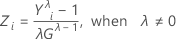0.5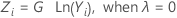0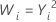−0.5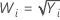−1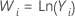## Determining optimal λ

Optimal λ is the value between −5 and 5 that minimizes the standard deviation (σ) of the transformed data. In order to accurately compare σ for different values of λ, Minitab calculates standardized transformed values Zi for this comparison, according to the following formula:

To estimate σ for subgroups data, Minitab uses the unbiased estimate of the pooled standard deviation. To estimate σ for individuals data, Minitab uses the unbiased estimate of the average moving range.

## Notation

TermDescription
G geometric mean of the original data
By using this site you agree to the use of cookies for analytics and personalized content.  Read our policy